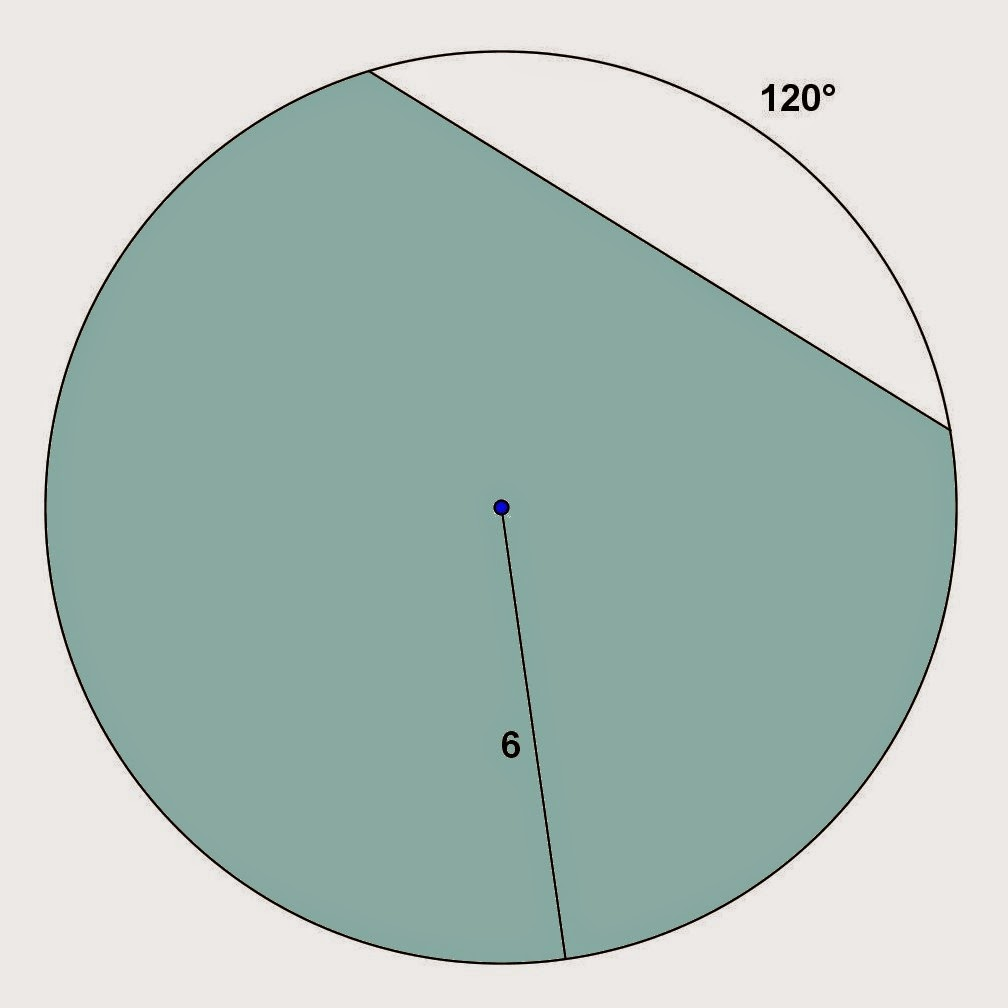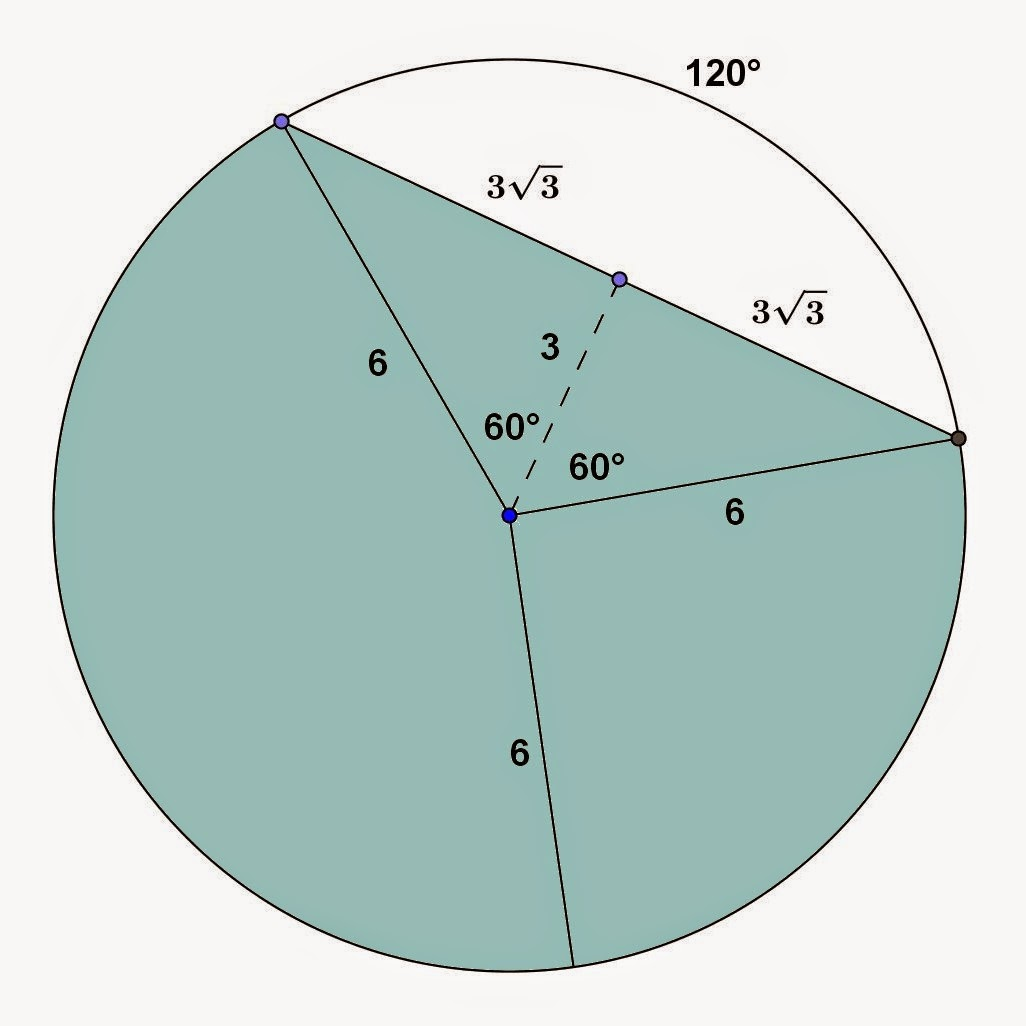## Monday, December 29, 2014

### Circular Segment Problems, 2

Category: Plane Geometry

"Published in Newark, California, USA"

Find the area of the shaded region:Photo by Math Principles in Everyday Life

Solution:

Consider the given figure abovePhoto by Math Principles in Everyday Life

The first thing that we need to do is to label further the given figure so that we can solve for the area of circular segment which is the unshaded portion as followsPhoto by Math Principles in Everyday Life

The angle of an arc is equal to the angle of circular sector which is 120°. If you draw a line which is perpendicular to the given chord, then it becomes two equal right triangles and that line will bisect the angle of circular sector. In this case, there are two 30° - 60° right triangles. Hence, the area of circular segment is

where θ is the angle of circular section in radians (a unit less value of angle). Substitute the values, we have

The area of a circle is

Therefore, the area of shaded region is

or